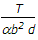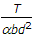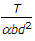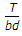# Civil Engineering - UPSC Civil Service Exam Questions

41.

A vertical photograph of a chimney was taken from an elevation of 500 m above M.S.L, The elevation of the base of the chimney was 250 m. If the relief displacement of the chimney was 51.4 mm and the radial distance of the image of the top of the chimney was 110 mm, the height of the chimney is

 A. 233.64 m B. 133.2 m C. 116.82 m D. 58.41m

Explanation:

No answer description available for this question. Let us discuss.

42.

A beam of rectangular cross-section (b x d) is subjected to a torque T. What is the maximum torsional stress induced in the beam ?(Where b < d, and α is a constant)

 A.B.C.D.Explanation:

No answer description available for this question. Let us discuss.

43.

A simply supported beam is subjected to an eccentric concentrated load. Where does the maximum deflection of the beam due to the applied load occur ?

 A. Directly under the load B. At the centre of the beam C. Between the load point and the nearest end support D. Between the load point and centre of the beam.

Explanation:

No answer description available for this question. Let us discuss.

44.

Which of the following factors are taken into account for estimating the runway length required for aircraft landing
1. Normal maximum temperature.
2. Air port elevation.
3. Maximum lending weight.
Select the correct answer using the codes given below:

 A. 1, 2, 3 and 4 B. 1, 3 and 4 C. 2 and 3 D. 1, 2 and 4

Explanation:

No answer description available for this question. Let us discuss.

45.

Two geometrically similar pumps are running at the same speed of 1000 r.p.m. and lifting water against the heads of 25 m and 16 m respectively. First pump is having an impeller diameter of 300 mm. The impeller diameter of second pump shall be

 A. 192 mm B. 240 mm C. 300 mm D. 469 mm